Next: Discussion Up: FMRI data Previous: Methods

## Results

Figures 9 and 10 show the results of inferring on the three different continuous weights mixture models we have described on the visual paradigm and audio paradigm SPMs respectively. The spatial maps in the figures are unthresholded marginal posterior means of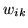, i.e.for all three classes of deactivation, non-activation and activation. Interestingly, the audio dataset shows a large amount of deactivation. If we qualitatively consider the data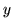shown in the top left of figure 10 then the spatial pattern of deactivation would seem to be strongly supported. Model 2, with the spatial smoothness parameter set to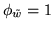imposes too much spatial smoothness. Indeed, if we look at the adaptively determined spatial smoothness in the box plot of figure 8, then the MRF smoothness parameter,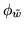, for the visual and audio datasets is far less than the fixed value ofused for model 2.

Figures 11 and 12 show maps of the weights for the activation class,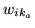, thresholded to leave only those voxels with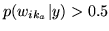for the visual paradigm and audio paradigm SPMs respectively. The choice of threshold on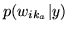is, as with any thresholding, a decision that needs to be made by the experimenter. However, this is the only time that any value in the inference of the model has to be chosen. Indeed, even when we choose the threshold for, there is a natural choice to make. That is we can choose the threshold of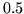which gives us an equal loss function where the chance of a false positive is equal to the chance of a false negative.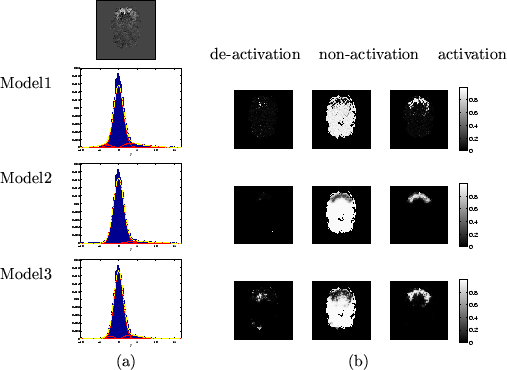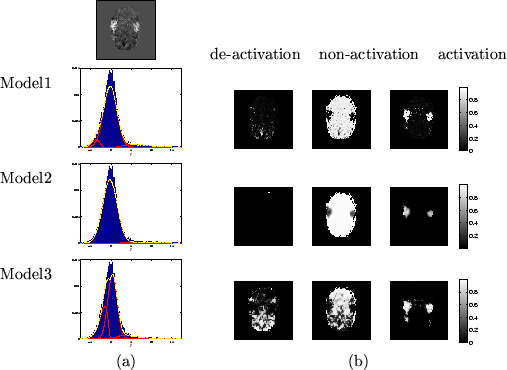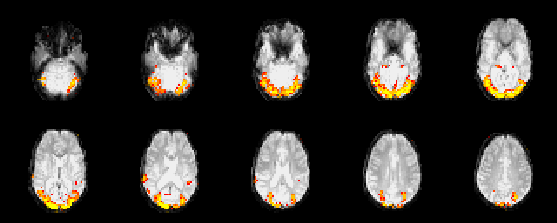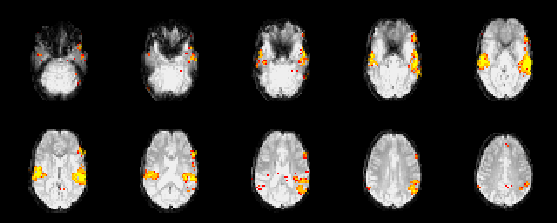Next: Discussion Up: FMRI data Previous: Methods